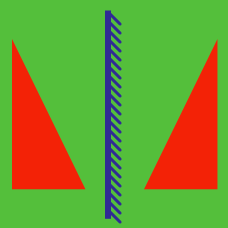Geometry

# Translation

Point $(2, 1)$ is moved to point $(10,11)$ by $T: (x, y) \rightarrow (x+a, y+b).$ What is $a+b$?

If we translate the point $(9, 3)$ by $(13, 2),$ what would be the new point?

The curve $y=2x^2+3x-9$ undergoes a parallel translation by $7$ units in the positive direction of the $x$-axis and $-3$ units in the positive direction of the $y$-axis. If the equation of the resulting curve is $y=ax^2+bx+c,$ what is $a+b+c?$

Consider a translation of axes $T: (x, y) \to (x+a, y+b)$ such that the coordinates of a point $P=(7, 4)$ with respect to the new system of coordinates after translation are $P'=(10,-11).$ What is the value of $a+b?$

If the point $(m,n)$ is shifted by $6$ in the positive $x$ direction and by $-12$ in the positive $y$ direction, then it coincides with the center of the circle $x^2+y^2-6x+2y-13=0.$ What is the value of $m+n?$

×